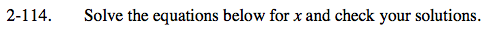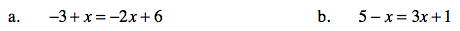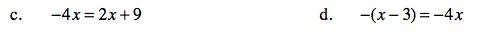### Home > CAAC > Chapter 2 > Lesson 2.2.3 > Problem2-114

2-114.
1. Solve the equations below for x and check your solutions. Homework Help ✎

1. −3 + x = −2x + 6

2. 5 − x = 3x + 1

3. −4x = 2x + 9

4. −(x − 3) = −4xIsolate the variable on one side of the equation and solve for x.

x = 1 Show your work.

Use the same strategy as in part (a).Use the same strategy as in part (a).

x = −1.5 Show your work.

Start by distributing the negative sign, then use the same strategy as in part (a).